# How to Adjust Axle Wheel Alignment for Scania L Series Truck

This instruction show you guide on how to adjust axle wheel alignment for Scania L series truck.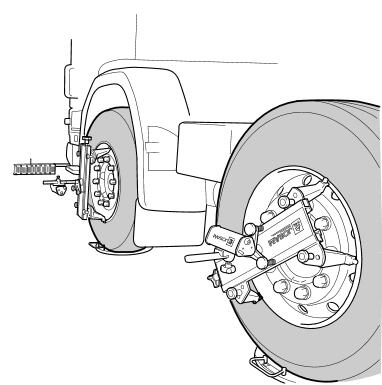Related Contents:

Procedures:

If two laser heads are available, it is beneficial to have both fitted. Otherwise, the laser head must be moved between the axles.

IMPORTANT!

Measurements should be taken on the linkage side (always left) with the engine running.

1.Fit the measuring equipment. See Before work – Preparations.

2.See the illustration for the measuring scale designation.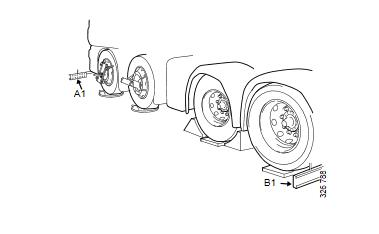3.Place the left-hand wheel of the first axle in the straight-ahead position. Direct the laser beam alternately on the measuring scales of A1 and B1. Turn the wheels until the same value is obtained on both measuring scales. Enter the value in the test report, e.g. 164. C1-ZERO=164.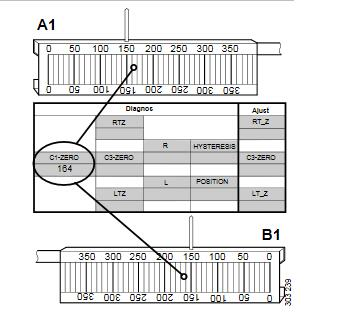4.Place the left-hand wheel of the second axle in the straight-ahead position. Direct the laser beam alternately on the measuring scales of A1 and B1. Turn the wheels until the same value is obtained on both measuring scales. Enter the value in the test report, e.g. 168. C3-ZERO=168.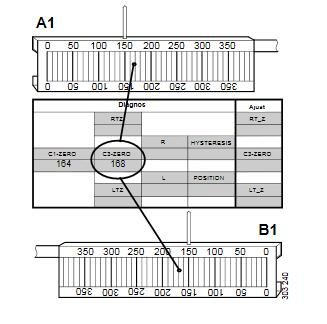5.Turn the steering wheel approximately half a turn to the right and then return it to the straight-ahead position for the first axle.

6.Take a reading for the second axle at B1 and enter the value in the test report, e.g. 182. RTZ=182.7.Turn the steering wheel approximately half a turn to the left and then return it to the straight-ahead position for the first axle.

8.Take a reading for the second axle at B1 and enter the value in the test report, e.g. 210. LTZ=210.Calculating axle alignment

1.Calculate the value for the R box. R = C3-ZERO – RTZ, 168 – 182 = -14. Enter the value -14 in the R box.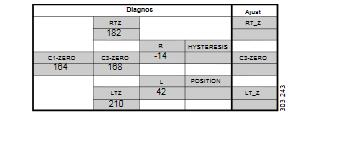2.Calculate the value for the L box. L = LTZ – C3-ZERO, 210 – 168 = 42. Enter the value 42 in the L box.

3.Calculate the value for the HYSTERESIS box.

HYSTERESIS = (R + L)/2, (-14 + 42)/2 = 14. Enter the value 14 in the HYSTERESIS box.

HYSTERESIS represents the play in joints and the elasticity in links and stays, which is distributed in both directions from the straight-ahead position.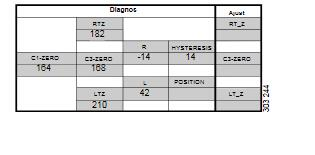4.Calculate the value for the POSITION box.

POSITION = R – L, -14 – 42 = -56. Enter the value -56 in the POSITION box.

POSITION represents the position of the play on the left-hand wheel of the second axle in relation to the left-hand wheel of the first axle. If the value is zero it means that the second axle is correctly adjusted and the play is evenly distributed to the right and left with the axle in the straight-ahead position.

A positive value means that the second axle is pulling to the right.

A negative value means that the second axle is pulling to the left.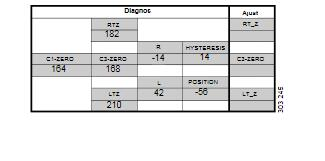1.Calculate the adjustment values for ADJUST. Transfer the value for C3-ZERO to ADJUST. Enter the value 168 in the C3-ZERO box.

RT_Z = C3-ZERO – HYSTERESIS, 168 – 14 = 154. Enter the value 154 in the RT_Z box.

LT_Z = C3-ZERO + HYSTERESIS, 168 + 14 = 182. Enter the value 182 in the LT_Z box.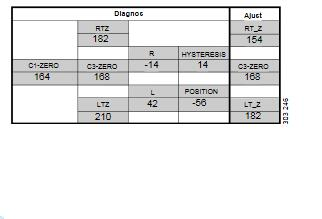2.When the POSITION value is positive, adjust in position RT_Z.

3.Turn the steering wheel approximately half a turn to the right and then return it to the straight-ahead position for the first axle according to C1-ZERO, 164 on B1.

4.Shorten the draglink until the value for ADJUST RT_Z 154 is obtained.

5.When the POSITION value is negative, adjust in position LT_Z.

6.Turn the steering wheel approximately half a turn to the left and then return it to the straight-ahead position for the first axle according to C1-ZERO, 164 on B1.

7.Lengthen the draglink until the value for ADJUST LT_Z 182 is obtained.

Adjustment has been carried out. If necessary, recheck the wheel alignment.

(Visited 12 times, 1 visits today)